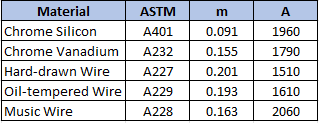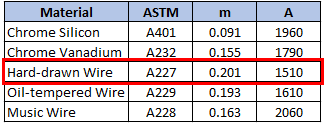## Torsional Stress

A hard-drawn wire with a diameter of 5 cm will be used as a spring material in a static assembly. Approximate the max allowable torsional stress for the wire.Hint
The max allowable torsional stress for cold-drawn carbon steel in static applications can be approximated as:
$$S_{sy}=\tau = 0.45\times S_{ut}$$$Hint 2 For spring materials, the minimum tensile strength for common spring steels can be determined from: $$S_{ut}=\frac{A}{d^{m}}$$$
where $$S_{ut}$$ is the tensile strength in MPa, and $$d$$ is the wire diameter in millimeters.
The max allowable torsional stress for cold-drawn carbon steel (ASTM A227, A228, & A229) in static applications can be approximated as:
$$S_{sy}=\tau = 0.45\times S_{ut}$$$For spring materials, the minimum tensile strength for common spring steels can be determined from: $$S_{ut}=\frac{A}{d^{m}}$$$
where $$S_{ut}$$ is the tensile strength in MPa, $$d$$ is the wire diameter in millimeters, and $$A$$ and $$m$$ can be found in the below table:Since the problem is asking for a hard-drawn wire:
$$S_{ut}=\frac{1510}{(50mm)^{0.201}}=\frac{1510}{2.195}=687.83\: MPa$$$Thus, the max allowable torsional stress for a hard-drawn wire is approximately: $$\tau = 0.45\times (687.83MPa)=310\:MPa$$$
310 MPa